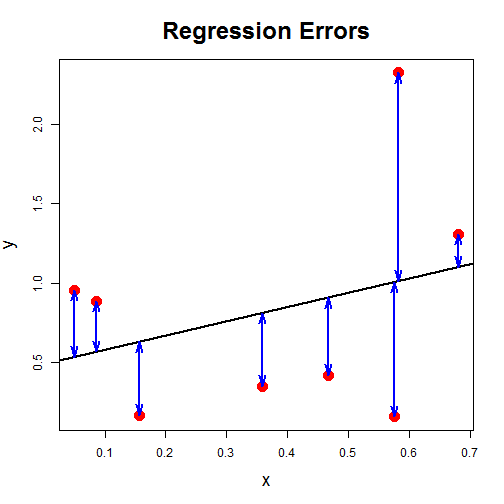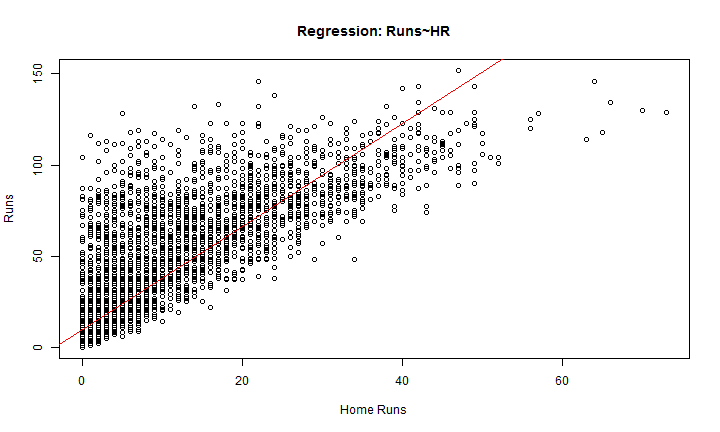# Data Analysis III: Simple Regression

SE350 Team

### Correlation

Correlation measures association or dependency quantitatively

$r=\frac{1}{(n-1)}\sum\limits_{i=1}^{n}\left( \frac{(x_i-\bar{x})}{s_x}\right)\left( \frac{(y_i-\bar{y})}{s_y}\right)$

• Between -1 and 1
• $$R >0$$ indicates positive relationship
• $$R<0$$ indicates negative relationship
• When r is close to 1 or negative 1, the association is strong, but close to 0 indicates a weak association

use the CORREL() command in Excel or the cor(x,y) command in R

### Regression Analysis

The process of using data to formulate relationships is known as regression analysis.

• Response Variable: the variable that will be predicted by the values of other variables
• Explanatory Variables: These variables can explain quantitatively, at least in part, the values of the the response variable.
• Simple Regressions: One explanatory variable (this lesson)
• Multiple Regressions: More than one explanatory variable (next lesson)

Regressions models can fall into two other categories: Linear (SE350) or Nonlinear (Not SE350).

### Understanding Regression:

Regression is a means to find the line that most closely matches the observed relationship between x and y. $y=a+bx+e$

Most common approach is the sum of squared differences between the observed and model values. This is what is going on “underneath the hood” of your computer when you do regression:

$e_i=y_i-y=y_i-(a+bx_i)$ $SS=\sum\limits_{i=1}^{n}e_{i}^{2}=\sum\limits_{i=1}^{n}(y_i-a-bx_i)^2$

### Picturing Regression

All that this equation is doing is placing the linear line in such a way that it minimizes the sum of the square of the error lengths:### Example: Build Regression Model

Let's import and subset our baseball data again:

bb<-read.csv("baseball.csv",as.is=TRUE)  ##Import Data
bb2<-subset(bb,year>1986)   ##Subset Data


Now let's build a linear model between

bb.lm<-lm(r~hr,data=bb2)


### Example: Model Summary

summary(bb.lm)


Call:
lm(formula = r ~ hr, data = bb2)

Residuals:
Min     1Q Median     3Q    Max
-87.36  -9.68  -7.68   4.86 104.16

Coefficients:
Estimate Std. Error t value Pr(>|t|)
(Intercept)   9.6794     0.2838    34.1   <2e-16 ***
hr            2.8312     0.0234   120.9   <2e-16 ***
---
Signif. codes:  0 '***' 0.001 '**' 0.01 '*' 0.05 '.' 0.1 ' ' 1

Residual standard error: 18.4 on 5901 degrees of freedom
Multiple R-squared:  0.712, Adjusted R-squared:  0.712
F-statistic: 1.46e+04 on 1 and 5901 DF,  p-value: <2e-16


### Understanding the Summary:

• $$R^2$$: Measures the percent of variation in the response variable accounted for by the regression model (note that this is the square of correlation)
• F-statistic and its significance: How likely is it that we would get the $$R^2$$ value that we observed if ALL of the true regression coefficients were zero?
• Adjusted $$R^2$$: This is another form of $$R^2$$ that has been adjusted if there are more than one explanatory variable. It is only considered in mutiple variable regression (next lesson).
• p-statistic (or p-value): How likely is it that we would get an estimate of the regression coefficient at least this large if the true value of the regression coefficient were zero?

### Example: Model Plot

plot(bb2$hr,bb2$r, xlab="Home Runs",ylab="Runs", main="Regression: Runs~HR")
abline(bb.lm,col="red")• Run regression of Hits~Runs. Plot it. Run a Summary. Does this improve your model?
• Run regression of On-Base~Runs. On Base = Hits + Base on Balls + Hit by Pitch. Plot this new model. Run a Summary. Does this improve your model?

### Improving our Model-Hits (1 of 3)

bb.lm2<-lm(r~h,data=bb2)
summary(bb.lm2)


Call:
lm(formula = r ~ h, data = bb2)

Residuals:
Min     1Q Median     3Q    Max
-35.79  -2.61   0.83   1.27  56.65

Coefficients:
Estimate Std. Error t value Pr(>|t|)
(Intercept) -0.83179    0.14430   -5.76  8.6e-09 ***
h            0.54212    0.00178  305.41  < 2e-16 ***
---
Signif. codes:  0 '***' 0.001 '**' 0.01 '*' 0.05 '.' 0.1 ' ' 1

Residual standard error: 8.37 on 5901 degrees of freedom
Multiple R-squared:  0.94,  Adjusted R-squared:  0.94
F-statistic: 9.33e+04 on 1 and 5901 DF,  p-value: <2e-16


### Improving our Model-On Base (2 of 3)

Let's try to calculate the number of time the player gets on base. This is hits+base on ball+hit by pitch. This is calculated and modeled below:

bb2$ob<-bb2$h+bb2$bb+bb2$hbp
bb.lm3<-lm(r~ob,data=bb2)


### Improving our Model-On Base (3 of 3)

summary(bb.lm3)


Call:
lm(formula = r ~ ob, data = bb2)

Residuals:
Min     1Q Median     3Q    Max
-38.13  -2.23   0.92   1.35  37.87

Coefficients:
Estimate Std. Error t value Pr(>|t|)
(Intercept) -0.916526   0.115329   -7.95  2.3e-15 ***
ob           0.380942   0.000989  385.09  < 2e-16 ***
---
Signif. codes:  0 '***' 0.001 '**' 0.01 '*' 0.05 '.' 0.1 ' ' 1

Residual standard error: 6.71 on 5900 degrees of freedom
(1 observation deleted due to missingness)
Multiple R-squared:  0.962, Adjusted R-squared:  0.962
F-statistic: 1.48e+05 on 1 and 5900 DF,  p-value: <2e-16


### Summary of Data (1 of 2):

This data frame contains batting statistics for a subset of players collected from http://www.baseball-databank.org/. There are a total of 21,699 records, covering 1,228 players from 1871 to 2007. Only players with more than 15 seasons of play are included.

Name Description
id unique player id
year year of data
team team played for
lg league
g number of games
ab number of times at bat
r number of runs
h hits

### Summary of Data (2 of 2):

Name Description
X2b hits on which the batter reached second base safely
X3b hits on which the batter reached third base safely
hr number of home runs
rbi runs batted in
sb stolen bases
cs caught stealing
bb base on balls (walk)
so strike outs
ibb intentional base on balls
hbp hits by pitch
sh sacrifice hits
sf sacrifice flies
gidp ground into double play